### Displayed Output

The output from the LP procedure is discussed in the following six sections:

For integer-constrained problems, the procedure also displays an Integer Iteration Log. The description of this Log can be found in the section Integer Programming. When you request that the tableau be displayed, the procedure displays the Current Tableau. The description of this can be found in the section The Reduced Costs, Dual Activities, and Current Tableau.

A problem data set can contain a set of constraints with several right-hand sides and several objective functions. PROC LP considers each combination of right-hand side and objective function as defining a new linear programming problem and solves each, performing all specified sensitivity analysis on each problem. For each problem defined, PROC LP displays a new sequence of output sections. Example 5.1 in the section Examples: LP Procedure discusses each of these elements.

The LP procedure produces the following displayed output by default.

#### The Problem Summary

The problem summary includes the

• type of optimization and the name of the objective row (as identified by the ID or ROW variable)

• name of the SAS variable that contains the right-hand-side constants

• name of the SAS variable that contains the type keywords

• density of the coefficient matrix (the ratio of the number of nonzero elements to the number of total elements) after the slack and surplus variables have been appended

• number of each type of variable in the mathematical program

• number of each type of constraint in the mathematical program

#### The Solution Summary

The solution summary includes the

• termination status of the procedure

• objective value of the current solution

• number of phase 1 iterations that were completed

• number of phase 2 iterations that were completed

• number of phase 3 iterations that were completed

• number of integer iterations that were completed

• number of integer feasible solutions that were found

• number of initial basic feasible variables identified

• time used in solving the problem excluding reading the data and displaying the solution

• number of inversions of the basis matrix

• current value of several of the options

#### The Variable Summary

The variable summary includes the

• column number associated with each structural or logical variable in the problem

• name of each structural or logical variable in the problem. (PROC LP gives the logical variables the name of the constraint ID. If no ID variable is specified, the procedure names the logical variable _OBSn_, whereis the observation that describes the constraint.)

• variable’s status in the current solution. The status can be BASIC, DEGEN, ALTER, blank, LOWBD, or UPPBD, depending upon whether the variable is a basic variable, a degenerate variable (that is, a basic variable whose activity is at its input lower bound), a nonbasic variable that can be brought into the basis to define an alternate optimal solution, a nonbasic variable at its default lower bound 0, a nonbasic variable at its lower bound, or a nonbasic variable at its upper bound.

• type of variable (whether it is logical or structural, and, if structural, its bound type, or other value restriction). See Example 5.1 for a list of possible types in the variable summary.

• value of the objective coefficient associated with each variable

• activity of the variable in the current solution

• variable’s reduced cost in the current solution

#### The Constraint Summary

The constraint summary includes the

• constraint row number and its ID

• kind of constraint (whether it is an OBJECTIVE, LE, EQ, GE, RANGELE, RANGEEQ, RANGEGE, or FREE row)

• number of the slack or surplus variable associated with the constraint row

• value of the right-hand-side constant associated with the constraint row

• current activity of the row (excluding logical variables)

• current activity of the dual variable (shadow price) associated with the constraint row

#### The Infeasible Information Summary

The infeasible information summary includes the

• name of the infeasible row or variable

• current activity for the row or variable

• type of the row or variable

• value of right-hand-side constant

• name of each nonzero and nonmissing variable in the row

• activity and upper and lower bounds for the variable

#### The RHS Sensitivity Analysis Summary

The RHS sensitivity analysis summary includes the

• value of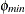• leaving variable when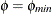• objective value when• value of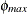• leaving variable when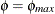• objective value when• column number and name of each logical and structural variable

• variable’s status when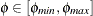• variable’s reduced cost when• value of right-hand-side constant when• activity of the variable when• value of right-hand-side constant when• activity of the variable when#### The Price Sensitivity Analysis Summary

The price sensitivity analysis summary includes the

• value of• entering variable when• objective value when• value of• entering variable when• objective value when• column number and name of each logical and structural variable

• variable’s status when• activity of the variable when• price of the variable when• variable’s reduced cost when• price of the variable when• variable’s reduced cost when